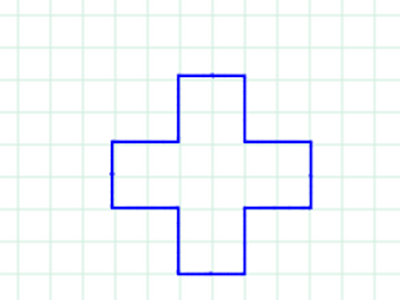## Monday, October 19, 2015

### Loop-de-loops

Like many mathy families and classes in the past couple of weeks, we've been playing with Anna Weltman's Loop-de-Loop patterns.

Note: to give credit where it is due, my introduction came via Mike Lawler's blog that linked with Dan Meyer's blog.

## Our first loops-de-loop

To start us out, I drew one example, then gave two different sequences to J1 and J2 to draw: (3, 7, 6) and (3, 1, 4).

As you can see from the picture, that led quickly to their own questions:
1. What if we did (3, 4, 1) instead of (3, 1, 4)?
2. What if we had four numbers in our sequence instead of 3? Their first test was  (1, 1, 2, 2)
3. How can we figure out where we should start drawing? This was a pressing problem, since we had fairly course graph paper (the grid was hand made!)

## A tool

Ahead of introducing this to the J's, I had made a short pencilcode program to automate drawing the loops-de-loop: Draw a rectangular loop-de-loop with your choice of step lengths

This let us easily try many different combinations of step lengths and build up our menagerie very quickly. As usual, the kids came up with ideas that wouldn't have occurred to me. These are behind some of the challenges below.

## Some challenges

For each of the following, what sequence of numbers defines the loop-de-loop pattern we created?Challenge 1: a basic l-d-lChallenge 2: test your assumptions about loops-de-loopChallenge 3: Where's my 4-fold symmetry?!Challenge 4: Whoa! Do we turn clockwise sometimes?

## Our favorite, so far

#### 1 comment:

1.Some natural ideas for how to use loops-de-loop in a coding class:
1) basic program to draw a static loop-de-loop

2) program that generates a random loop-de-loop (maybe within some constrained family)

3) draw a user-input loop-de-loop

4) draw a non-rectangular l-d-l (interesting to think about what constraints you keep)

5) draw l-d-l based on some well-known sequence (for example, digits of e, pi)

6) automate the coloring of the regions of the l-d-l